# Transforming Atari 2600 Controller

A modern replacement of an Atari controller that transforms between a joystick and a paddle using the same base.

IntermediateFull instructions provided8 hours2,989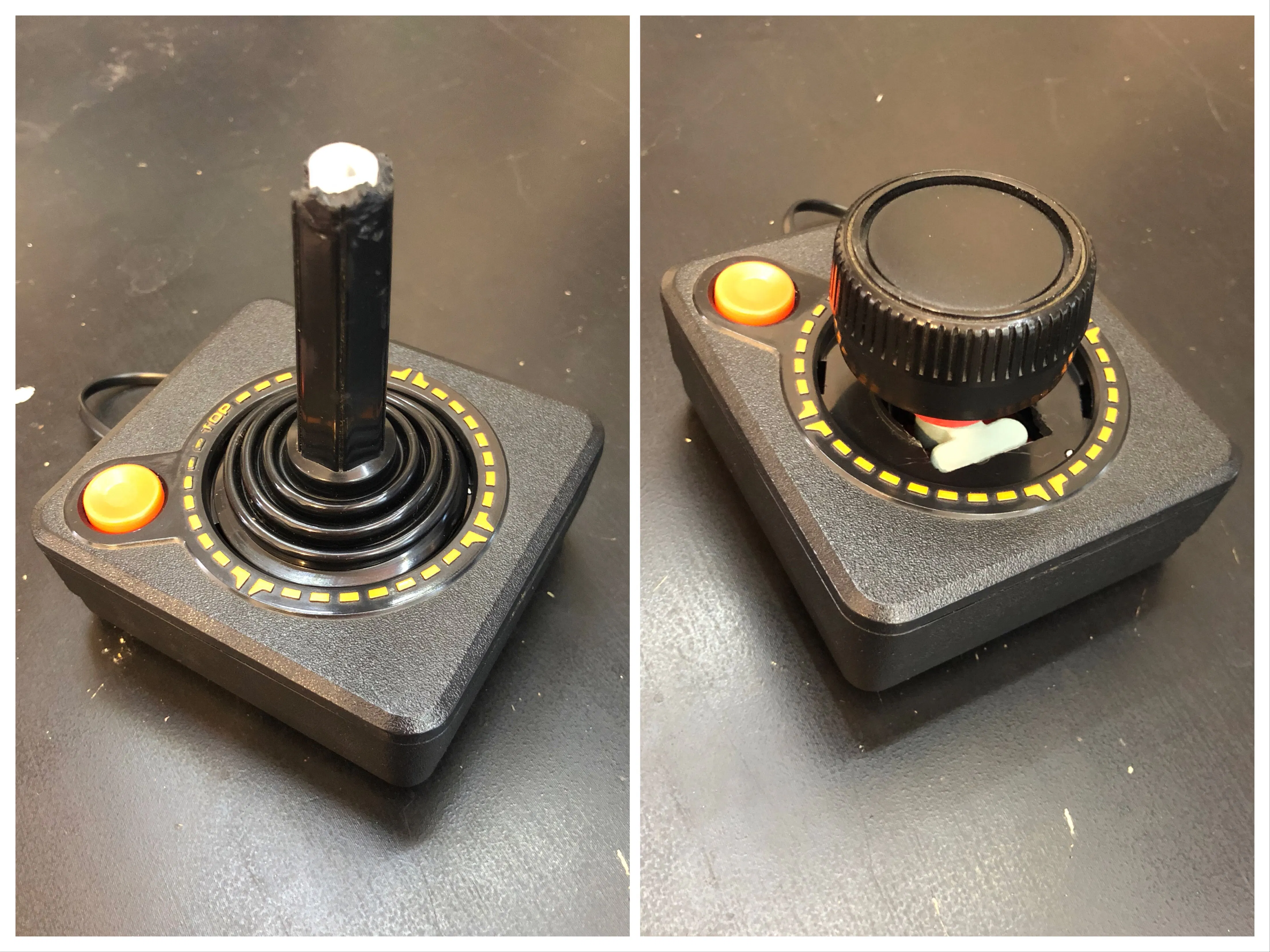## Things used in this project

### Hardware components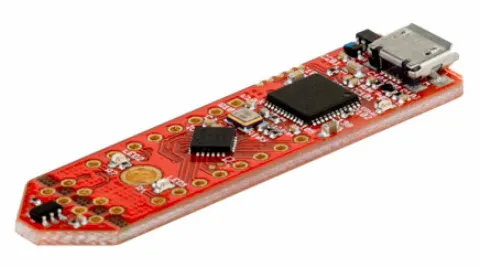Infineon 3D Magnetic Sensor 2Go
×1
 Infineon Joystick for 3D 2Go
×1
 Infineon Rotate Knob for 3D 2Go
×1Resistor 10k ohm
×6Resistor 1k ohm
×3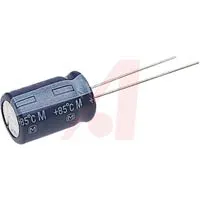Capacitor 10 µF
×1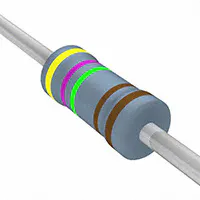Resistor 4.75k ohm
×1
 STMicroelectronics TSV911
×1SparkFun Pushbutton switch 12mm
×1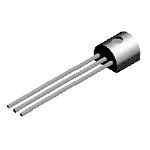General Purpose Transistor PNP
×1
 Resistor 27k ohm
×1
 Diode - 1N4001
×8
 DB9 female solder cup connector
×1
 DB9 male to female cable
×1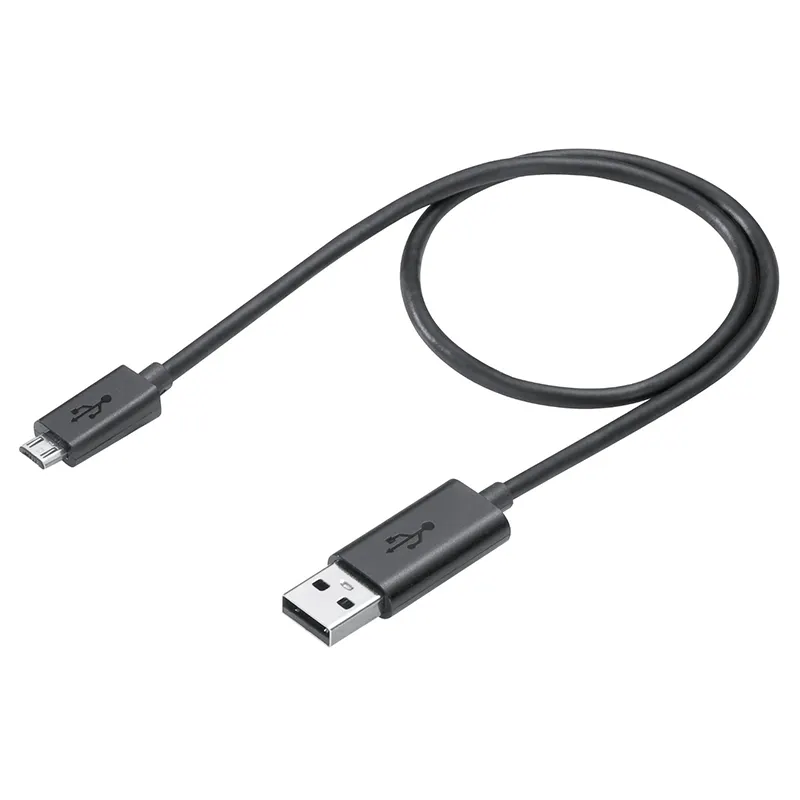Micro-USB to USB Cable (Generic)
×1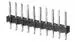Male Header 40 Position 1 Row (0.1")
×1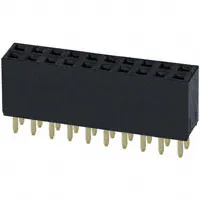Female Header 20 Position 2 Row (0.1")
×1

### Software apps and online servicesArduino IDE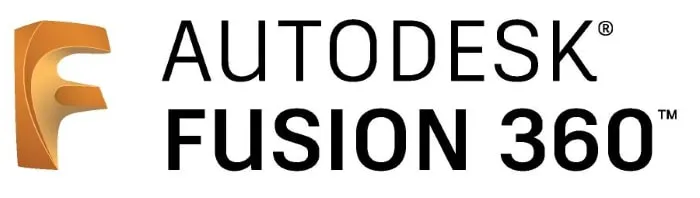Autodesk Fusion 360

### Hand tools and fabrication machines3D Printer (generic)Soldering iron (generic)

## Schematics

### Schematic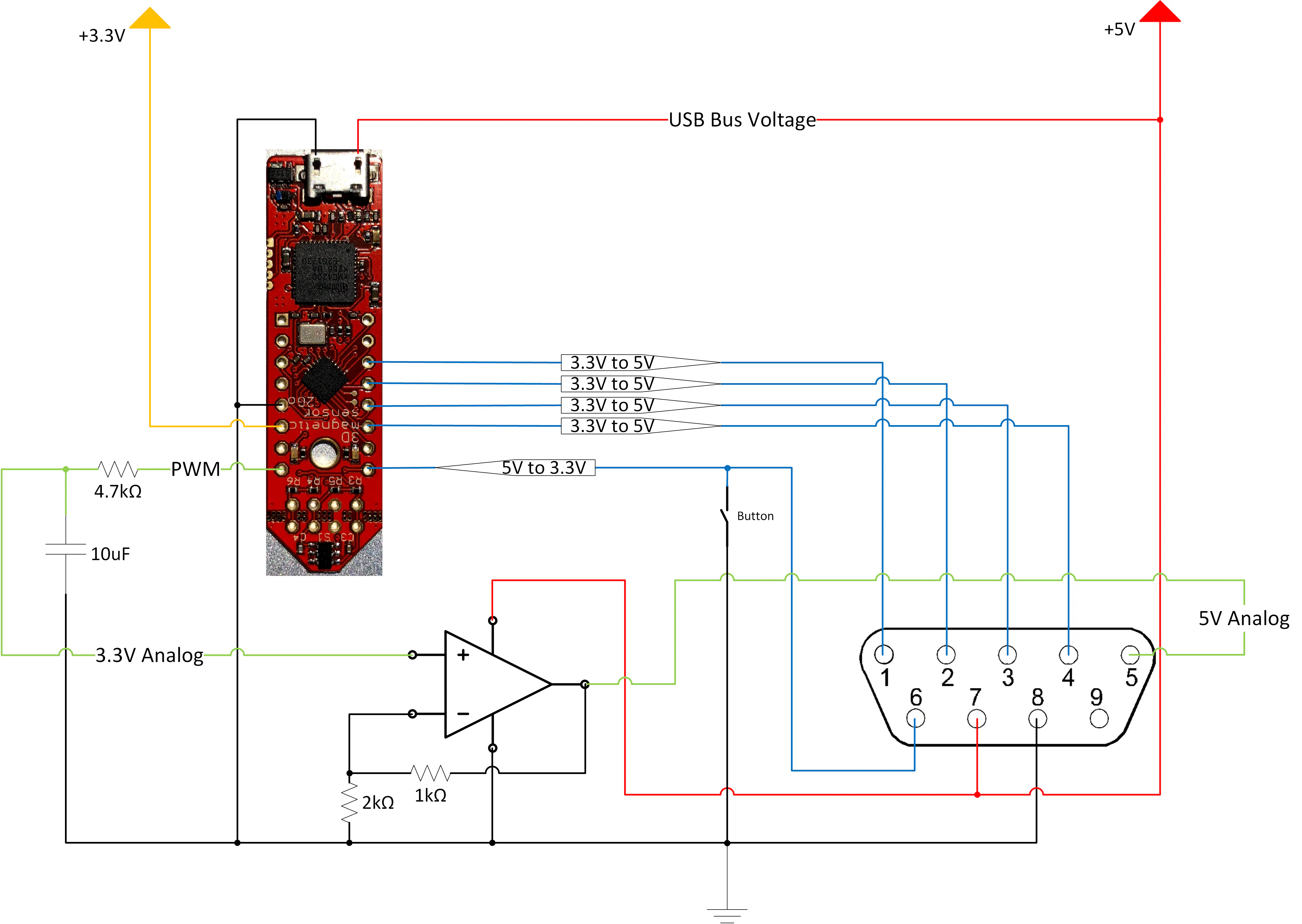### 3.3V to 5V translation subcircuit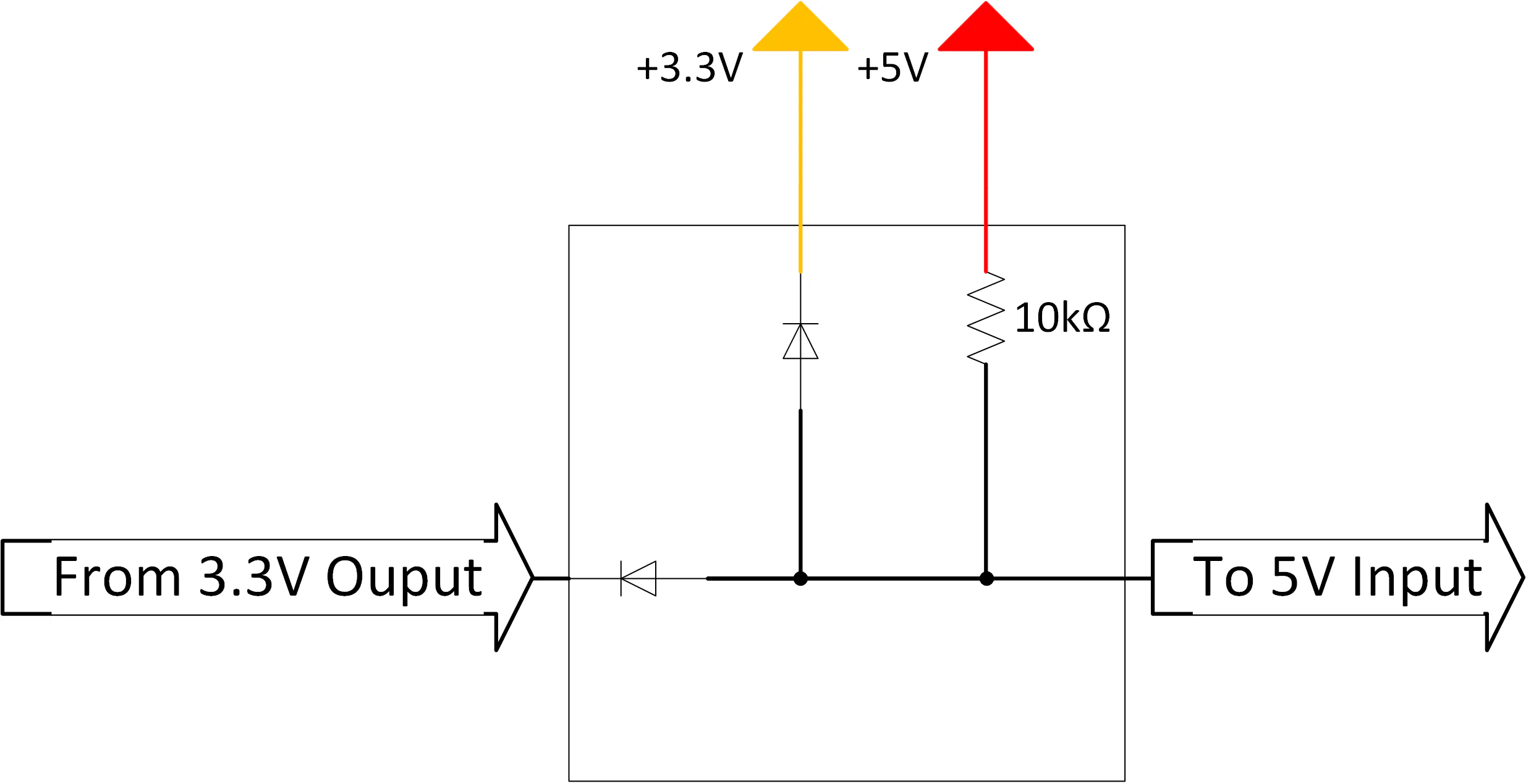### 5V to 3.3V translation subcircuit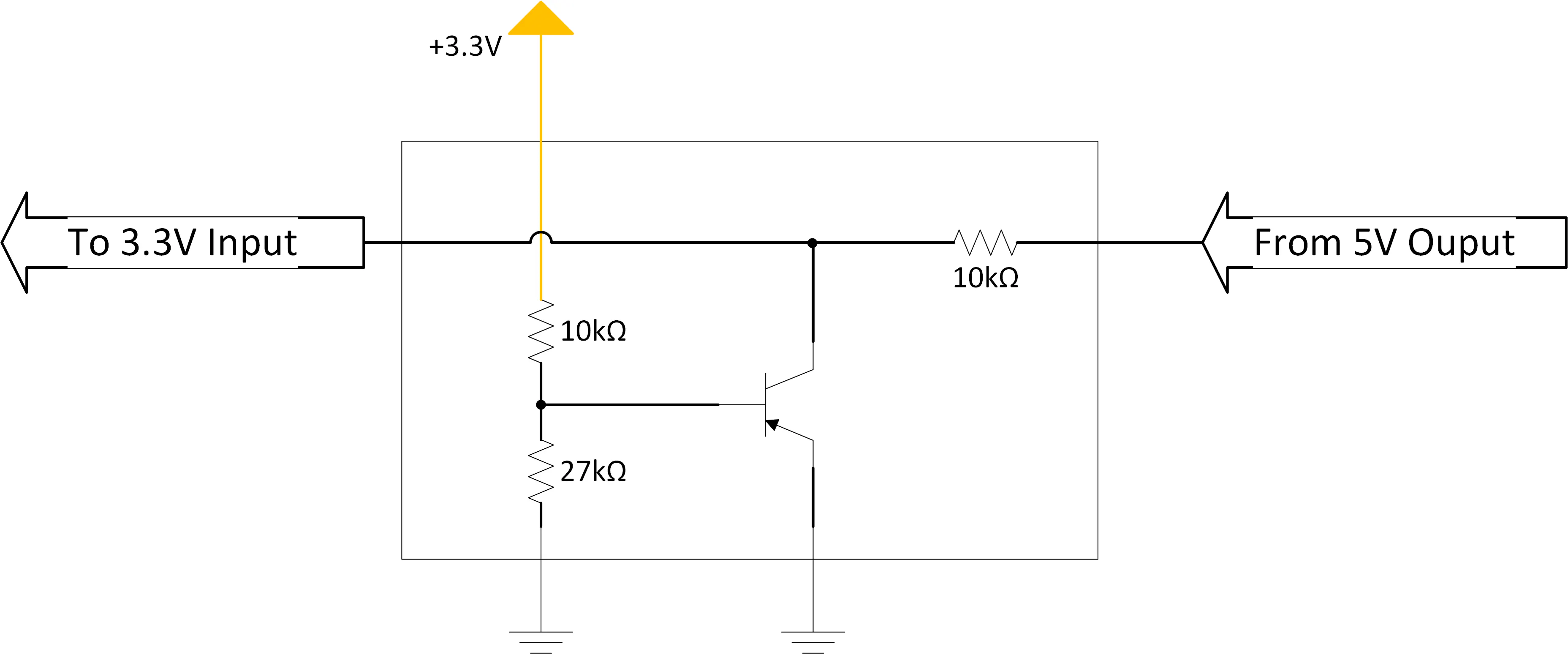## Code

### Transforming Atari Controller

Arduino
Code for Arduino IDE. More details in Code section.
```#include <Tle493d_w2b6.h>

// directions for joystick
int up = 5;     // P0.15
int down = 4;   // P0.14
int left = 3;   // P0.9
int right = 2;  // P0.8

// button (originally) for joystick
int button = 1; // P0.6

int rightPot = 8; // P0.5 (PWM pin)

// (uses same pin as right on joystick)
int rightButton = right;  // P0.8

// set up sensor
Tle493d_w2b6 Tle493dMagnetic3DSensor = Tle493d_w2b6();

// hold controller modes
typedef enum
{
JOYSTICK,
} ContollerModeTypeDef;

ContollerModeTypeDef controllerMode;

// limits for joystick measurements on my controller
#define x_max 7.15
#define x_min -8.84
#define y_max 10.0
#define y_min -8.5

void detectControllerMode(void)
{
// if button is held, go into paddle mode
{
}
// else go into joystick mode
else{
controllerMode = JOYSTICK;
}
}

// when the joystick is in the center, set all directions high (disabled)
{
digitalWrite(up, HIGH);
digitalWrite(down, HIGH);
digitalWrite(left, HIGH);
digitalWrite(right, HIGH);

//Serial.print(" nothing");
}

void setup()
{
// Need to start, reset, then start again to deal with power up glitches
Tle493dMagnetic3DSensor.begin();
Tle493dMagnetic3DSensor.resetSensor();
Tle493dMagnetic3DSensor.begin();

//Serial.begin(9600);
//while (!Serial);

// joystick directions are outputs
pinMode(up, OUTPUT);
pinMode(down, OUTPUT);
pinMode(left, OUTPUT);
pinMode(right, OUTPUT);

// default joystick outputs as high

pinMode(rightPot, OUTPUT);
pinMode(rightButton, OUTPUT);

// set paddle button as high (released)
digitalWrite(rightButton, HIGH);

// button is input to detect controller mode
pinMode(button, INPUT);

// detect if controller should act as joystick or paddle
detectControllerMode();
}

void loop() {
{
// store angle of knob from last loop to determine which way it moved
float angle_prev = 0;

// the length of the 500Hz period that is high
// 255 is the max
int dutyCycle = 255/2;

// the new loop
while(1)
{
// get new data
Tle493dMagnetic3DSensor.updateData();

// get X and Y positions for rotation calculation
float x = Tle493dMagnetic3DSensor.getX();
float y = Tle493dMagnetic3DSensor.getY();

float angle = atan2(y,x);
//Serial.print(angle);

// calculate difference
float diff = angle_prev - angle;

//Serial.print(",");
//Serial.print(diff);

// a factor which stores the direction we're spinning
int direction = 0;

// increasing (cw)
if (diff > 0.01)
{
direction = 3;
}
// wrapping around (cw)
else if (diff < -1.00)
{
direction = 1;
}
// decreasing (ccw)
else if (diff < -0.01)
{
direction = -3;
}
// wrapping around (ccw)
else if (diff > 1.00)
{
direction = -1;
}
// not moving
else
{
direction = 0;
}

//Serial.print(",");
//Serial.print(direction);

// add the direction to the duty cycle
dutyCycle += direction;

// duty cycle bounds checking
if (dutyCycle < 0)
{
dutyCycle = 0;
}
if (dutyCycle > 255)
{
dutyCycle = 255;
}

// write position (dutyCycle) to pin as PWM
// at 500 Hz
// so use RC filter with
// R = 4.7k
// C = 0.1uF
// to convert to analog voltage
analogWrite(rightPot, dutyCycle);

//Serial.print(",");
//Serial.print(dutyCycle);

// save angle for next round
angle_prev = angle;

// measure paddle's distance and press button if near
int norm = Tle493dMagnetic3DSensor.getNorm();
//Serial.println(norm);

// go low if either button is pressed
int buttonState = HIGH;

// ~20 when released
// ~55 when pressed
// use midway (42) as threshold
if (norm > 42)
{
// pressed
buttonState =  LOW;
//Serial.print(",");
//Serial.println(LOW);
}

// also check if joystick's button is pressed
{
buttonState = LOW;
}

// set button output if either button is pressed
digitalWrite(rightButton, buttonState);
}
}
if (controllerMode == JOYSTICK)
{
// store how far the direction is to the limit
// as a percentage
float up_pct = 0;
float down_pct = 0;
float left_pct = 0;
float right_pct = 0;

// the new loop
while(1)
{
// get new data
Tle493dMagnetic3DSensor.updateData();

// get X and Y positions
float x = Tle493dMagnetic3DSensor.getX();
float y = Tle493dMagnetic3DSensor.getY();

//Serial.print(x);
//Serial.print(",");
//Serial.println(y);
//Serial.print(",");

// get percentages by dividing current position by limit
up_pct = x/x_max;
// x is around -3.3 when neutral so add that term back in
down_pct = (x + 3.3)/x_min;
left_pct =  y/y_max;
right_pct  = y/y_min;

// Go through each possible direction and the one with the
// highest percentage is the chosen direction
// Check that the percentage is >25% before setting else
// you're in the deadzone so disable all direction pins
// (just to be safe)
if (up_pct > down_pct)
{
if (up_pct > left_pct)
{
if (up_pct > right_pct)
{
if(up_pct > 0.25)
{
//Serial.print(up_pct);
//Serial.print(", up");
digitalWrite(up, LOW);
}
}
else
{
if(right_pct > 0.25)
{
//Serial.print(right_pct);
//Serial.print(", right");
digitalWrite(right, LOW);
}
}
}
else {
if(left_pct > 0.25)
{
//Serial.print(left_pct);
//Serial.print(", left");
digitalWrite(left, LOW);
}
}
}
else {
if (down_pct > left_pct)
{
if (down_pct > right_pct)
{
if(down_pct > 0.25)
{
//Serial.print(down_pct);
//Serial.print(", down");
digitalWrite(down, LOW);
}
}
else
{
if(right_pct > 0.25)
{
//Serial.print(right_pct);
//Serial.print(", right");
digitalWrite(right, LOW);
}
}
}
else {
if(left_pct > 0.25)
{
//Serial.print(left_pct);
//Serial.print(", left");
digitalWrite(left, LOW);
}
}
}
}
}
}
```

## Credits

### Dillon Nichols

8 projects • 17 followers
Electrical engineer: hardware/firmware; tinkerer; hobbyist; amateur fabricator;